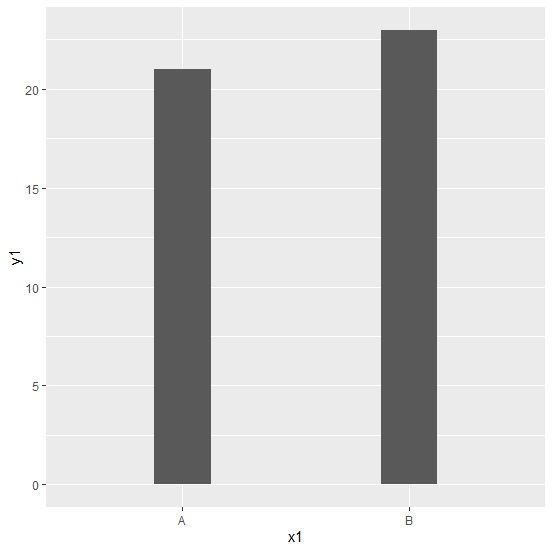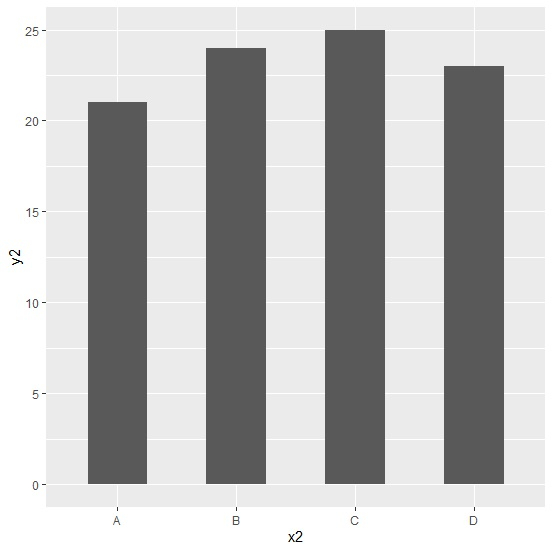# How to create multiple bar plots for varying categories with same width bars using ggplot2 in R?

To create multiple bar plots for varying categories with same width bars using ggplot2, we would need to play with width argument inside geom_bar function to match the width of the bars in each bar plot. The best way to do this would be setting the larger ones to 0.25 and the shorter ones to 0.50.

## Example

Consider the below data frame −

Live Demo

x1<-c("A","B")
y1<-c(21,23)
df1<-data.frame(x1,y1)
df1

## Output

   x1  y1
1  A   21
2  B   23

Loading ggplot2 package and creating bar plot for categories in x1 −

## Example

library(ggplot2)
plot1<-ggplot(df1,aes(x1,y1))+geom_bar(stat="identity",width=0.25)
plot1

## OutputConsider another data frame with more categories −

x2<-c("A","B","C","D")
y2<-c(21,24,25,23)
df2<-data.frame(x2,y2)

Creating the bar plot for categories in x2 that matches the width of the bars in plot1 −

## Example

plot2<-ggplot(df2,aes(x2,y2))+geom_bar(stat="identity",width=0.5)
plot2

## Output